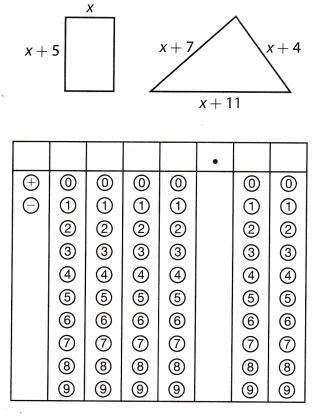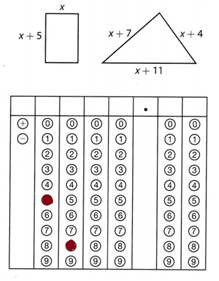Refer to our Texas Go Math Grade 8 Answer Key Pdf to score good marks in the exams. Test yourself by practicing the problems from Texas Go Math Grade 8 Module 11 Quiz Answer Key.

11.1 Equations with the Variable on Both Sides

Solve.

Question 1.
4a – 4 = 8 + a ____________
4a – 4 = 8 + a
(Subtract a from both sides.) 4a – 4 – a = 8 + a – a
(Add 4 to both sides.) 3a – 4 = 8
(Divide both sides by 3.) 3a = 12
a = 4
The statement is true. There is one solution.

Question 2.
4x + 5 = x + 8 ___________
4x + 5 = x + 8
(Subtract x from both sides) 4x + 5 – x = x + 8 – x
(Subtract 5 from both sides.) 3x + 5 = 8
(Divide both sides by 3.) 3x = 3
x = 1
The statement is true. There is one solution.

Question 3.
Hue is arranging chairs. She can form 6 rows of a given length with 3 chairs left over, or 8 rows of that same length if she gets 11 more chairs. Write and solve an equation to find how many chairs are in that row length.
(Write an equation) 6x + 3 = 8x – 11
(Subtract 3 from both sides.) 6x + 3 – 3 = 8x – 11 – 3
(Subtract 8x from both sides.) 6x = 8x – 14
(Divide by -2.)  – 2x = -14
x = 7
There are 7 chairs in each row.

11.2 Equations with Rational Numbers

Solve.

Question 4.
$$\frac{2}{3}$$s – $$\frac{2}{3}$$ = $$\frac{s}{6}$$ + $$\frac{4}{3}$$ ____________
(Multiply both sides by the LCM(3, 6) = 6) $$\frac{2}{3}$$s – $$\frac{2}{3}$$ = $$\frac{s}{6}$$ + $$\frac{4}{3}$$
6 ∙ $$\frac{2}{3}$$s – 6 ∙ $$\frac{2}{3}$$ = 6 ∙ $$\frac{s}{6}$$ + 6 ∙ $$\frac{4}{3}$$
4s – 4 = n + 8
(Add 4 to both sides) 4s – 4 + 4 = s + 8 + 4
4s = s + 12
(Subtract n from both sides) 4s – s = s + 12 – s
3s = 12
(Divide both sides by 3) $$\frac{3s}{3}$$ = $$\frac{12}{3}$$
s = 4

Question 5.
1.5d + 3.25 = 1 + 2.25d _____________
1.5d + 3.25 = 1 + 2.25d
(Subtract 3.25 from both sides) 1.5d + 3.25 – 3.25 = 1 + 2.25d – 3.25
1.5d = 2.25d – 2.25
(Subtract 2.25d from both sides.) 1.5d – 2.25d = 2.25d – 2.25 – 2.25d
-0.75d = -2.25
(Divide both sides by -0.75) $$\frac{-0.75d}{-0.75}$$ = $$\frac{-2.25}{-0.75}$$
d = 3

Question 6.
Happy Paws charges $19.00 plus$1.50 per hour to keep a dog during the day. Woof Watchers charges $15.00 plus$2.75 per hour. Write and solve an equation to find for how many hours the total cost of the services is equal.
Happy Paws charge for x hours
1.5x + 19
Woof Watchers charge for x hours
2.75x + 15
Put two expressions as equal
2.75x + 15 = 1.5x + 19
Subtract 1.5 from both sides
2.75x + 15 – 1.5x = 1.5x + 19 – 1.5x
1.25x + 15 = 19
Subtract 15 from both sides
1.25x + 15 – 15 = 19 – 15
1.25x = 4
Divide both sides by 1.25
x = $$\frac{4}{1.25}$$ = 3.2
The total cost of the services is equal after $3.2 hrs. 11.3 inequalities with the Variable on Both Sides Question 7. Write an inequality to represent the relationship “Two less than 2 times a number is greater than the number plus 64″. Then solve your inequality. Answer: Let us consider the number = x 2 – 2x ≥ x + 60 -2x -x ≥ 60 – 2 -3x ≥ 58 x ≥ 58/-3 x ≥ -19.33 2 – 2(-19.33) ≥ -19.33 + 60 40.66 ≥ 40.67. 11.4 Inequalities with Rational Numbers Question 8. One prepaid cell phone company charges$0.028 per minute and a $3 monthly fee. Another company charges$0.034 per minute with no monthly fee. For what numbers of minutes per month are the charges for the first company cheaper?
Let us consider the number of charges = x
The first company charges = $0.028 and$3 for the monthly fee.
The secondary company charges = $0.034 and no monthly fee. The inequality equation is$0.029 + $3 ≤$0.034x
Multiply both sides by 1000
28x + 2000 ≤ 34x
2000 ≤ 34x + 28x
2000 ≤ 62x
x ≥ 2000/62
X ≥ 32.2
32.2 minutes per month are the charges for the first company cheaper.

Essential Question

Question 9.
How can you use equations with the variable on both sides to solve real-world problems?
I could join a game club for $15 and rent games for$2 each, or I could pay $3 for each video rental, which is the better deal? Texas Go Math Grade 8 Module 11 Mixed Review Texas Test Prep Answer Key Selected Response Question 1. Two cars are traveling in the same direction. The first car is going 40 mi/h and the second car is going 55 mi/h. The first car left 3 hours before the second car. Which equation could you solve to find how many hours it will take for the second car to catch up to the first car? (A) 55t + 3 = 40t (B) 55t + 165 = 40t (C) 40t + 3 = 55t (D) 40t + 120 = 55t Answer: (D) 40t + 120 = 55t Explanation: We write an expression representing the distance that car A has traveled. Let’s denote the time by t. 3 * 40 + 40t = 120 + 40t We write an expression representing the distance that car B has traveled. Let’s denote the time by t 55t We write an equation that can be solved to find the time it will take for the second car to catch up to the first car. 40t + 120 = 55t Question 2. A wide screen television display measures approximately 15 inches high and 27 inches wide. A television is advertised by giving the approximate length of the diagonal of its screen. How should the television be advertised? (A) 36 inches (B) 31 inches (C) 30 inches (D) 21 inches Answer: The wide screen television display is in the shape of rectangle The formula for the length of the diagonal of a rectangle = c² = a² + b² The length of the rectangle = a = 15 Wide of the rectangle = b = 27 c² = 15² + 27² c² = 225 + 729 c = sq. root (954) c = 31 sq. inches. Option B is the correct answer. Question 3. Shawn’s Rentals charges$27.50 per hour to rent a surfboard and a wet suit. Darla’s Surf Shop charges $23.25 per hour to rent a surfboard plus$17 extra for a wetsuit. For what total number of hours are the charges for Shawn’s Rentals the same as the charges for Darla’s Surf Shop?
(A) 3
(B) 4
(C) 5
(D) 6
(B) 4

Explanation:
Shawn’s rentaL charge after x hrs
27.5x
Darlas surf shop charge after x hr
23.25x + 17
Equate the two expressions
23.25x + 17 = 27.5x
Subtract 23.25x from both sides
23.25x + 17 – 23.25x = 27.5x – 23.25x
4.25x = 17
Divide both sides by 4.25
x = $$\frac{17}{4.25}$$ = 4
The charge would be equal after \$4 hrs.

Question 4.
Which of the following is an irrational number?
(A) -8
(B) 4.63
(C) $$\sqrt{11}$$
(D) $$\frac{1}{3}$$
(C) $$\sqrt{11}$$

Explanation:
$$\sqrt{11}$$ is irrational.

Question 5.
Carlos has at least as many action figures in his collection as Josh. Carlos has 5 complete sets plus 4 individual figures. Josh has 3 complete sets plus 14 individual figures. Which inequality represents how many action figures can be in a complete set?
(A) x ≥ 7
(B) x ≤ 7
(C) x ≥ 5
(D) x ≤ 5
Let us consider the action figures in a complete set = x
The Charles has 5 complete sets + 4 individual figures
Josh has 3 complete sets + 14 individual figures
The inequality equation is
5x + 4 ≤ 3x + 14
5x – 3x ≤ 14 – 4
2x ≤ 10
x ≤ 10/2
x ≤ 5
Option D is the correct answer.

Question 6.
Which inequality represents the solution to 1.25x + 2.5 < 2.75x – 6.5?
(A) x > 6
(B) x < 6
(C) x > 2.25
(D) x < 2.25
1.25x + 2.5 < 2.75x – 6.5
1.25x – 2.75x < -6.5 – 2.5
-1.50x < -9
1.5x < 9
x < 9/1.5
x < 6
Option B is the correct answer.

Gridded Response

Question 7.
If both figures have the same perimeter, what is the perimeter of each figure?Given that,
Perimeter of the rectangle = perimeter of the triangle
2(l + w) = a + b + c
l = x + 5
w = x
a = x + 7
b = x + 4
c = x + 11
2(x + 5 + x) = x + 7 + x + 4 + x + 11
4x + 10 = 3x + 22
22 – 10 = 4x – 3x
x = 12.
3(12) + 22 = 4(12) + 10
58 = 58
The perimeter of the rectangle is 58 sq. inches.
The perimeter of the triangle is 58 sq. inches.Scroll to Top